## Example Questions

← Previous 1

### Example Question #21 : Coordinate Geometry

In the xy -plane, line is given by the equation 2x - 3y = 5. If line passes through the point (a ,1), what is the value of a ?

-2
5
-1
4
3
Explanation:

The equation of line l relates x -values and y -values that lie along the line. The question is asking for the x -value of a point on the line whose y -value is 1, so we are looking for the x -value on the line when the y-value is 1. In the equation of the line, plug 1 in for and solve for x:

2x - 3(1) = 5

2x - 3 = 5

2x = 8

x = 4. So the missing x-value on line l is 4.

### Example Question #22 : Coordinate Geometry

The equation of a line is: 2x + 9y = 71

Which of these points is on that line?

(2,7)

(4,-7)

(4,7)

(-4,7)

(-2,7)

(4,7)

Explanation:

Test the difference combinations out starting with the most repeated number.  In this case, y = 7 appears most often in the answers.  Plug in y=7 and solve for x.  If the answer does not appear on the list, solve for the next most common coordinate.

2(x) + 9(7) = 71

2x + 63 = 71

2x = 8

x = 4

Therefore the answer is (4, 7)

### Example Question #1 : How To Find Out If A Point Is On A Line With An Equation

Which of the following lines contains the point (8, 9)?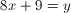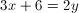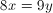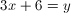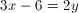Explanation:

In order to find out which of these lines is correct, we simply plug in the values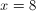and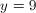into each equation and see if it balances.

The only one for which this will work is### Example Question #473 : Geometry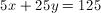Which point lies on this line?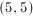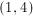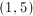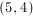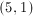Explanation:Test the coordinates to find the ordered pair that makes the equation of the line true: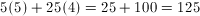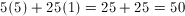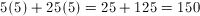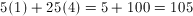### Example Question #2 : How To Find Out If A Point Is On A Line With An Equation

Consider the lines described by the following two equations:

4y = 3x2

3y = 4x2

Find the vertical distance between the two lines at the points where x = 6.

12

48

44

21

36

21

Explanation:

Since the vertical coordinates of each point are given by y, solve each equation for y and plug in 6 for x, as follows:Taking the difference of the resulting -values give the vertical distance between the points (6,27) and (6,48), which is 21.

### Example Question #52 : Coordinate Geometry

For the line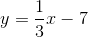Which one of these coordinates can be found on the line?

(3, 7)

(3, 6)

(6, 12)

(6, 5)

(9, 5)

(3, 6)

Explanation:

To test the coordinates, plug the x-coordinate into the line equation and solve for y.

y = 1/3x -7

Test (3,-6)

y = 1/3(3) – 7 = 1 – 7 = -6   YES!

Test (3,7)

y = 1/3(3) – 7 = 1 – 7 = -6  NO

Test (6,-12)

y = 1/3(6) – 7 = 2 – 7 = -5  NO

Test (6,5)

y = 1/3(6) – 7 = 2 – 7 = -5  NO

Test (9,5)

y = 1/3(9) – 7 = 3 – 7 = -4  NO

### Example Question #53 : Coordinate Geometry

Solve the following system of equations:

–2x + 3y = 10

2x + 5y = 6

(3, 5)

(2, 2)

(–2, –2)

(3, –2)

(–2, 2)

(–2, 2)

Explanation:

Since we have –2x and +2x in the equations, it makes sense to add the equations together to give 8y = 16 yielding y = 2.  Then we substitute y = 2 into one of the original equations to get x = –2.  So the solution to the system of equations is (–2, 2)

### Example Question #22 : Other Lines

Which of the following sets of coordinates are on the line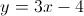?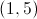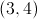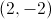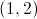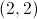Explanation:when plugged in for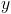andmake the linear equation true, therefore those coordinates fall on that line.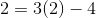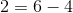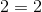Because this equation is true, the point must lie on the line. The other given answer choices do not result in true equalities.

### Example Question #55 : Coordinate Geometry

Which of the following points can be found on the line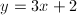?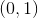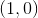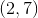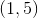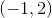Explanation:

We are looking for an ordered pair that makes the given equation true. To solve, plug in the various answer choices to find the true equality.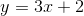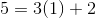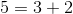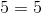Because this equality is true, we can conclude that the pointlies on this line. None of the other given answer options will result in a true equality.

### Example Question #482 : Geometry

Which of the following points is not on the line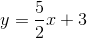?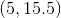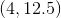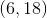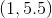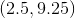Explanation:

To figure out if any of the points are on the line, substitute theandcoordinates into the equation. If the equation is incorrect, the point is not on the line. For the point: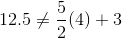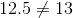So,is not on the line.

← Previous 1